# Trigonometric Series

Also found in: Dictionary, Thesaurus.
Related to Trigonometric Series: Fourier series

## trigonometric series

[¦trig·ə·nə¦me·trik ′sir·ēz]
(mathematics)
An infinite series of functions with n th term of the form an cos nx + bn sin nx.
McGraw-Hill Dictionary of Scientific & Technical Terms, 6E, Copyright © 2003 by The McGraw-Hill Companies, Inc.
The following article is from The Great Soviet Encyclopedia (1979). It might be outdated or ideologically biased.

## Trigonometric Series

an infinite series of functions of the form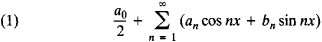that is, a series defined in terms of the sines and cosines of multiple angles. Trigonometric series are often written in the complex form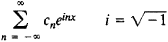The numbers an and bn or the numbers cn are called the coefficients of the trigonometric series.

Trigonometric series play an extremely important role in mathematics and its applications. First of all, trigonometric series provide a means of representing and studying functions and therefore are one of the main tools of the theory of functions. Furthermore, trigonometric series appear in a natural way in the solutions of a number of problems of mathematical physics—for example, the problem of the vibration of a string and the problem of the propagation of heat. Finally, the theory of trigonometric series has contributed to the refinement of such fundamental concepts of mathematical analysis as the function and the integral; has given rise to such important branches of mathematics as the theory of Fourier integrals and the theory of almost periodic functions; has provided one of the starting points for the development of set theory, the theory of functions of a real variable, and functional analysis; and has provided the basis for general harmonic analysis.

Trigonometric series first appear in L. Euler’s Introduction to the Analysis of Infinitesimals (1748) and in his letter to C. Gold-bach of July 4,1744—for example,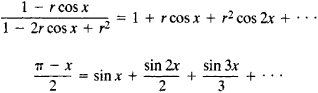Euler pointed out the following relation between power series and trigonometric series: if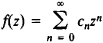where the cn are real, then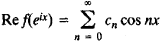where Re designates the real part of the function. Euler also was responsible for the first applications of trigonometric series to the vibrating-string problem (1748); in his opinion, only those functions that we now call piecewise analytic can be expanded in trigonometric series. With regard to the expansion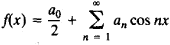the formulas for the coefficients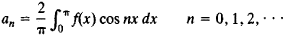were first given by A. Clairaut in 1757, and the derivation of the formulas by means of term-by-term integration of trigonometric series was presented by Euler in 1777. The formulas for a0 and a1, however, had been previously given by J. d’Alembert in 1754.

Trigonometric series attracted the interest of the most prominent mathematicians of the 1750’s to 1770’s in connection with the controversy over the vibrating-string problem. In particular, D. Bernoulli first advanced the assertion that an “arbitrary” function can be expanded in a trigonometric series. The concept of function, however, had not yet been sufficiently clearly defined.

The assertion that functions of an extremely general kind actually can be expanded in trigonometric series was again put forth in 1811 by J. Fourier, who persistently argued in support of the assertion. He systematically used trigonometric series in studying problems of heat conduction. An extremely broad class of trigonometric series has, with considerable justice, been named for him (Fourier series). After Fourier’s research, trigonometric series became an intrinsic part of mathematical physics; important contributions in this regard were made by S. Poisson and M. V. Ostrogradskii.

The substantial advances made in the theory of trigonometric series in the 19th century were due to the refinement of the basic concepts of mathematical analysis and the creation of the theory of functions of a real variable. Thus, P. Dirichlet in 1837, after defining more precisely the concept of arbitrary function, obtained the first general test for the convergence of Fourier series. As part of his work on trigonometric series, G. F. B. Riemann investigated the concept of integral and established the necessary and sufficient condition for the integrability of functions. Investigation of the representation of functions by trigonometric series led G. Cantor to the creation of set theory. Finally, between 1902 and 1906, H. Lebesgue applied the concepts of measure and integral he had developed to the theory of trigonometric series and gave the theory its modern form. Important contributions were made to the theory of trigonometric series by such Soviet mathematicians as N. N. Luzin and D. E. Men’shov.

### REFERENCES

Bari, N. K. Trigonometricheskie riady. Moscow, 1961.
Zygmund, A. Trigonometricheskie riady, 2nd ed., vols. 1–2. Moscow, 1965. (Translated from English.)
References in periodicals archive ?
Corduneanu, Formal trigonometric series, almost periodicity and oscillatory functions, Nonlinear Dynamics and Systems Theory, 13 (2013), 367-388.
Denote the partial sum of the trigonometric series as
Kozlov  were the first to construct some ordinary universal trigonometric series in the class of all measurable functions in the sense of a.e.
Since the shape is represented by trigonometric series, for through-wall shape reconstruction and wall parameters estimation, there are 10 parameters to be estimated by HMPPSO.
Concerning a sufficient condition of Sidon for the integrability of trigonometric series, Math.
The problem was solved repeatedly, with a different number of members of the truncated trigonometric series, which approximated radius vectors of separate objects.
The applied distributed load [q.sub.0] and [m.sub.0] are expanded in single trigonometric series as
The displacement solutions, however, are here expressed as an accelerated trigonometric series expansion, rather than a Fourier series supplemented by polynomials.
Taking account of the history of the field, he characterizes rigor as an explanation of the convergence of series in general and of the puzzling behavior of trigonometric series in particular.
As opposed to traditional formats, Bressoud (mathematics, Macalester College) starts with infinite series and concentrates on topics that revolve around questions raised by Fourier's trigonometric series and the restructuring of calculus.
 Bary N.K., 1964, A treatise on trigonometric series, Vol I & Vol II, Pergamon Press, London.
Developing into a single trigonometric series and by their adding coming up are to unique function (12) which determines the conditions at the edge x - [a.sub.1] of segment "1" of plate is obtained:

Site: Follow: Share:
Open / Close